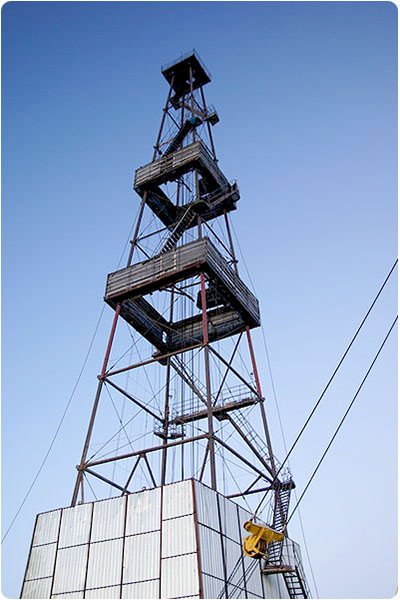# Ground electrode depth... from the book "Grounding: answers to questions"

...The information is the same everywhere, that the angles (vertical) must be 2.5 meters long. We have angles on metal base 6 meters long. I think to cut them in two cuts 2-meter each. And to hammer them in at the distance of 2 meters from each other.... Question. It is critical that they will be 2 meters instead of 2.5? Or necessarily 2.5 m?

Gray clay soil, always damp. Will I keep within the desired parameter limit (30 Ohms)?
========================================

Not critical :-) I don't understand where the length of 2.5 meters comes from... In fact, you can use 1-meter and 3-meter angles. And even 6 - if you can hammer them in :-)

Explanations and theory.

Grounding - is an electrical contact with the soil (ground). Is evaluated by the parameter = ground resistance. Ground resistance = resistance to current spreading from the electrode (ground electrode system) into the ground   + in the ground. It is a value showing how well the current diverges from the electric appliance to the ground in an emergency (or during the equipment operation). It is the value which estimats quality of the grounding.

Two factors have a major influence on it (grounding resistance):

1. Electrical soil resistivity in which the electrode is placed and in which the current from the electrode will "spread". The parameter estimating soil resistance to the passage of current.
2. Area of electrode's contact with the ground. The larger is the area - the "easier" is for the current to drain from the electrode into the ground.

Let's go back to your case.

Only the total area of the contact surface of the electrode (angle) with the ground is important. Wether you make 2 of them 3 meters each or 3 angles 2 meters each.

For more impact :-) Some calculations.

In your ground with an electrical resistivity of not more than 50 ohm * m - one electrode 2.5 meters deep will give ground resistance = 16 ohms. Three of these electrodes placed on the correct distance between each other (not less than the depth of immersion) will give 16/3 = 5.3 Ohm.

One electrode 2 meters deep will give 19 ohms. Three of these electrodes placed on the correct distance between each other (not less than the depth of immersion) will give 19/3 = 6.3 Ohm.

One electrode 1 meter deep will give 32 ohms. Three of these electrodes placed on the correct distance between each other (not less than the depth of immersion) will give 32/3 = 10.7 Ohm.

Even meter ground electrodes in the amount of 3 pieces will give you a lot lower ground resistance than necessary. That is really good.

However, we must not forget that in a fierce winter the ground freezes down to 1-1.5 meters, and then the largest part of the ground electrode system is below this mark.

Useful materials:

Related Articles: